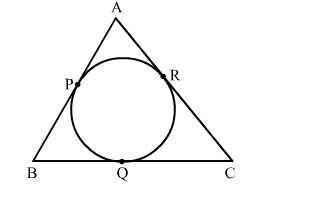# A circle is inscribed in ΔABC, touching AB, BC and AC at P, Q and R, respectively.Question:

A circle is inscribed in ΔABC, touching AB, BC and AC at PQ and R, respectively. If AB = 10 cm, AR = 7 cm and CR = 5 cm, find the length of BC.Solution:

Given, a circle inscribed in triangle $\mathrm{ABC}$, such that the circle touches the sides of the triangle at $\mathrm{P}, \mathrm{Q}, \mathrm{R}$.

Tangents drawn to a circle from an external point are equal.

$\therefore \mathrm{AP}=\mathrm{AR}=7 \mathrm{~cm}, \mathrm{CQ}=\mathrm{CR}=5 \mathrm{~cm} .$

Now, $B P=(A B-A P)=(10-7)=3 \mathrm{~cm}$

$\therefore B P=B Q=3 \mathrm{~cm}$

$\therefore B C=(B Q+Q C)$

$=>B C=3+5$

$\Rightarrow B C=8$

$\therefore$ The length of $B C$ is $8 \mathrm{~cm}$.